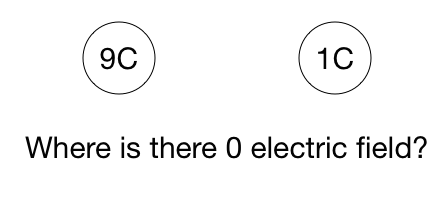# Vanishing Electric FieldTwo charges are on the $x$-axis. One of them is a $9\text{ C}$ charge located at $x = 0\text{ m}$. The other is a $1\text{ C}$ charge located at $x = 1\text{ m}$. Find the location on the $x$-axis where the net electric field due to these charges is $0 \text{ N/C}$.

×